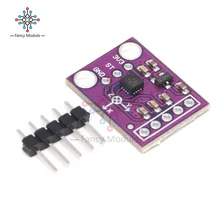# ADXL337 accelerometer Arduino Uno example

The ADXL337 is a small, thin, low power, complete 3-axis accelerometer with signal conditioned voltage outputs. The product measures acceleration with a minimum full-scale range of ±3g. It can measure the static acceleration of gravity in tiltsensing applications, as well as dynamic acceleration resulting from motion, shock, or vibration.The user selects the bandwidth of the accelerometer using the CX, CY, and CZ capacitors at the XOUT, YOUT, and ZOUTpins. Bandwidths can be selected to suit the application, with a range of 0.5 Hz to 1600 Hz for X and Y axes, and a range of 0.5 Hz to 550 Hz for the Z axis.

### Parts List

 Name Link Arduino Uno UNO R3 CH340G/ATmega328P, compatible for Arduino UNO ADXL337 3-axis ADXL337 GY-61 Replacement ADXL335 Module Analog Output Accelerometer Connecting wire Free shipping Dupont line 120pcs 20cm male to male + male to female and female to female jumper wire

### Code

No libraries required – this is a sparkfun example

 Source code```// Make sure these two variables are correct for your setup
int scale = 3; // 3 (±3g) for ADXL337, 200 (±200g) for ADXL377

void setup()
{
// Initialize serial communication at 115200 baud
Serial.begin(115200);
}

// Read, scale, and print accelerometer data
void loop()
{
// Get raw accelerometer data for each axis

// Scale map depends on if using a 5V or 3.3V microcontroller
float scaledX, scaledY, scaledZ; // Scaled values for each axis
scaledX = mapf(rawX, 0, 675, -scale, scale); // 3.3/5 * 1023 =~ 675
scaledY = mapf(rawY, 0, 675, -scale, scale);
scaledZ = mapf(rawZ, 0, 675, -scale, scale);

// Print out raw X,Y,Z accelerometer readings
Serial.print("X: "); Serial.println(rawX);
Serial.print("Y: "); Serial.println(rawY);
Serial.print("Z: "); Serial.println(rawZ);
Serial.println();

// Print out scaled X,Y,Z accelerometer readings
Serial.print("X: "); Serial.print(scaledX); Serial.println(" g");
Serial.print("Y: "); Serial.print(scaledY); Serial.println(" g");
Serial.print("Z: "); Serial.print(scaledZ); Serial.println(" g");
Serial.println();

delay(2000); // Minimum delay of 2 milliseconds between sensor reads (500 Hz)
}

// Same functionality as Arduino's standard map function, except using floats
float mapf(float x, float in_min, float in_max, float out_min, float out_max)
{
return (x - in_min) * (out_max - out_min) / (in_max - in_min) + out_min;
}```

### Output

Open the serial monitor and you will see something like this

X: 384
Y: 340
Z: 281

X: 0.41 g
Y: 0.02 g
Z: -0.50 g

X: 410
Y: 345
Z: 345

X: 0.64 g
Y: 0.07 g
Z: 0.07 g